### IMO Shortlist 2012 problem A6

Kvaliteta:
Avg: 0,0
Težina:
Avg: 8,0
Let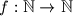$f: \mathbb{N} \rightarrow \mathbb{N}$ be a function, and let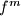$f^m$ be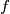$f$ applied$m$ times. Suppose that for every$n \in \mathbb{N}$ there exists a$k \in \mathbb{N}$ such that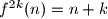$f^{2k}(n)=n+k$, and let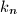$k_n$ be the smallest such$k$. Prove that the sequence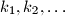$k_1,k_2,\ldots$ is unbounded.
Izvor: Međunarodna matematička olimpijada, shortlist 2012